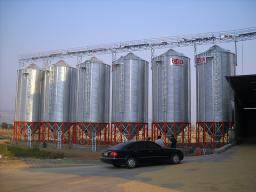# Hectoliters 3342

We filled the water tank from 3/5 of its volume. After releasing 21 hl, only 1/4 of the entire volume remained. How many hectoliters of water can the tank hold?

x =  36 hl

### Step-by-step explanation:

x = 3/5·V
x - 21 = 1/4·V

3V-5x = 0
V-4x = -84

Row 2 - 1/3 · Row 1 → Row 2
3V-5x = 0
-2.33x = -84

x = -84/-2.33333333 = 36
V = 0+5x/3 = 0+5 · 36/3 = 60

V = 60
x = 36

Our linear equations calculator calculates it.Did you find an error or inaccuracy? Feel free to write us. Thank you!

Tips for related online calculators
Do you have a linear equation or system of equations and looking for its solution? Or do you have a quadratic equation?